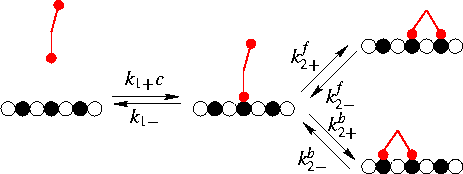Your browser can't run 1.1 Java applets.

n= average total number of heads per lattice site
n_1= average number of dimers bound with one head
n_d= average number dimers bound with both heads heads per lattice site

### Parameters

##### All reaction rates are given in inverse seconds
• k1+ c Association rate for the first head
• k2+b Association rate for the second head at the left of the first one
• k2+f Association rate for the second head at the right of the first one
• k2-b Dissociation rate for the left head of a double-bonded dimer
• k2-f Dissociation rate for the right head of a double-bonded dimer
• k1- Dissociation rate for a single-bonded dimer##### Dimensionless ratios:
K1=k1+/k1-
K2=k2+f/k2-f=k2+b/k2-b

### Predefined parameter sets

Three parameter sets showing interesting cases can be retrieved through buttons at the bottom. These include:
1. Irreversible decoration: No dissociation, binding of the second head on both sides much faster than the binding first one.
2. Explicit diffusion: K1=0.1, K2=1000, symmetric association of the second head
3. No explicit diffusion: K1=0.1, K2=1000, association of the second head only to one side

(C) Andrej Vilfan, Aug. 1999